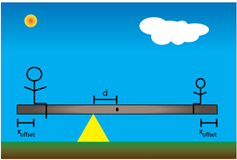# Problem: You have been hired to design a family-friendly see-saw. Your design will feature a uniform board (mass M= 11 kg: length L = 3.2 m) that can be moved so that the pivot is a distance d from the center of the board. This will allow riders to achieve static equilibrium even if they are of different mass, as most people are. You have decided that each rider will be positioned so that his/her center of mass will be a distance xoffset = 19 cm from the end of the board when seated as shown. You have selected a child of mass m1 = 23 kg (shown on the right), and an adult of mass m2 = 87 kg (shown on the left) to test out your prototype. Determine the distance d in meters. Determine the magnitude of the force exerted on the pivot point by the see-saw while in use in newtons.Part (a) Determine the distance d in metersPart (b) Determine the magnitude of the force exerted on the pivot point by the see-saw while in use in newtons

###### FREE Expert Solution

From the equilibrium condition of torques:

$\overline{){{\mathbf{m}}}_{{\mathbf{1}}}{\mathbf{g}}{\mathbf{\left(}}\frac{\mathbf{L}}{\mathbf{2}}{\mathbf{-}}{{\mathbf{x}}}_{\mathbf{o}\mathbf{f}\mathbf{f}\mathbf{s}\mathbf{e}\mathbf{t}}{\mathbf{+}}{\mathbf{d}}{\mathbf{\right)}}{\mathbf{+}}{\mathbf{M}}{\mathbf{g}}{\mathbf{\left(}}{\mathbf{d}}{\mathbf{\right)}}{\mathbf{=}}{{\mathbf{m}}}_{{\mathbf{2}}}{\mathbf{g}}{\mathbf{\left(}}\frac{\mathbf{L}}{\mathbf{2}}{\mathbf{-}}{{\mathbf{x}}}_{\mathbf{o}\mathbf{f}\mathbf{f}\mathbf{s}\mathbf{e}\mathbf{t}}{\mathbf{-}}{\mathbf{d}}{\mathbf{\right)}}}$

The magnitude of the force exerted at the pivot point is expressed as:

$\overline{){\mathbf{F}}{\mathbf{=}}{\mathbf{\left(}}{{\mathbf{m}}}_{{\mathbf{1}}}{\mathbf{+}}{{\mathbf{m}}}_{{\mathbf{2}}}{\mathbf{+}}{\mathbf{M}}{\mathbf{\right)}}{\mathbf{g}}}$

81% (430 ratings)###### Problem Details

You have been hired to design a family-friendly see-saw. Your design will feature a uniform board (mass M= 11 kg: length L = 3.2 m) that can be moved so that the pivot is a distance d from the center of the board. This will allow riders to achieve static equilibrium even if they are of different mass, as most people are. You have decided that each rider will be positioned so that his/her center of mass will be a distance xoffset = 19 cm from the end of the board when seated as shown. You have selected a child of mass m1 = 23 kg (shown on the right), and an adult of mass m2 = 87 kg (shown on the left) to test out your prototype. Determine the distance d in meters. Determine the magnitude of the force exerted on the pivot point by the see-saw while in use in newtons.Part (a) Determine the distance d in meters

Part (b) Determine the magnitude of the force exerted on the pivot point by the see-saw while in use in newtons